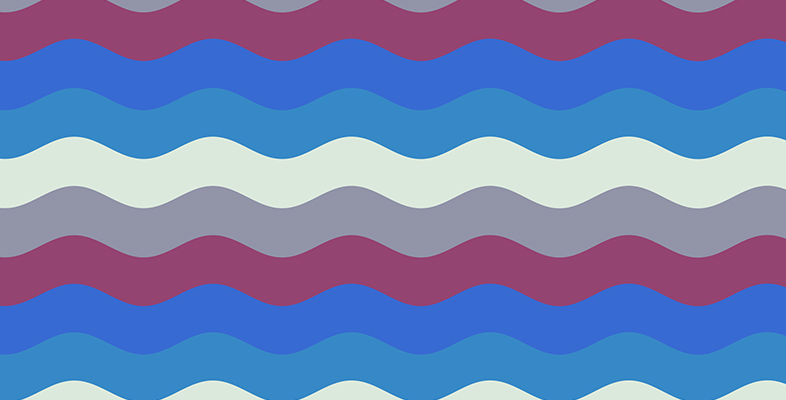Science, Maths & Technology

### Become an OU studentWhat are waves?

Start this free course now. Just create an account and sign in. Enrol and complete the course for a free statement of participation or digital badge if available.

# 2 Restoring forces

In our stone and water example, the water was initially at rest and at this point the upward and downward forces acting on it were in balance. From Newton’s laws, we know that when the forces are balanced there is no acceleration. However, the impact of the stone disturbs the natural level of the water surface, and because the water is displaced above or below the mean water level the forces are not in balance. It is now worth referring to Newton’s first law of motion which states that:

An object remains at rest or moves in a straight line at constant speed unless it is acted on by an unbalanced force.

Another way of writing this first law would be to say that an object does not accelerate unless it is acted on by an unbalanced force, or equivalently, if an object is acted on by an unbalanced force it will accelerate.

• When the water level is at its lowest point and the forces are most out of balance, in which direction is the force acting to restore the water surface to its mean level?

• The force is directed upwards as the surface of the water returns to the mean water level.

When the water level is at its maximum height there is a force directed downwards.

In both cases this force is called the restoring force.

Every time the surface reaches a maximum displacement a wave crest expands away from the point where the stone entered the water (Figure 1.3). The result is an increasing circle of ripples moving away from the initial disturbance. Eventually, as explained above, the ripples disappear.Figure 1 (repeated)  Ripples from a stone thrown in a pond.

Because the crests or the ‘maxima’ of the wave get smaller we say this example shows a damped wave.

• What would be the easiest way to make the circle of the expanding wave in Figure 1 larger?

• We could displace the surface by a greater amount by using a larger or heavier stone.

We could frame the whole cycle of what happened in Figure 1 as a system at rest (the flat-water surface) being disturbed (the stone being tossed into the water). Then a wave is generated as the system returns to rest once more.